Chapter Notes: Chemical Reactions & Equations

# Chemical Reactions & Equations Chapter Notes - Science Class 10

 Table of contentsWhat is Chemical Reaction?What is Chemical Equation?Balancing a Chemical EquationTypes of Chemical ReactionsOxidation and ReductionEffects of Oxidation Reaction on Everyday LifeFrequently Asked Questions (FAQs)Chemical Reactions and Chemical Equations are the foundational concept in the field of chemistry and play a critical role in our ability to understand and manipulate the natural world.
Overall, a deep understanding of chemical reactions and equations is essential for anyone seeking to develop new materials, improve existing processes, or gain insights into the behaviour of natural systems.
The study of chemical reactions and equations allows us to understand the properties and behaviour of substances at a molecular level.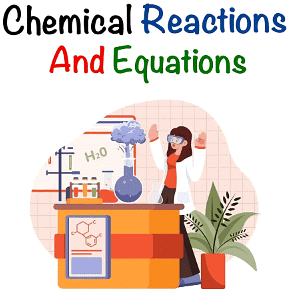By understanding chemical reactions and equations, we can predict the outcome of a reaction based on the properties of the reactants and the conditions under which the reaction occurs.

## What is Chemical Reaction?

A chemical reaction is a process in which one or more substances, the Reactants, are converted to one or more different substances, the Products.

Example: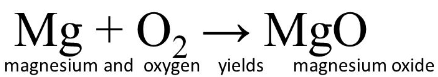The substances that undergo chemical change in the reaction, Magnesium and 0xygen, are the Reactants. The new substance, Magnesium Oxide, formed during the reaction, is the Product.

These are the following changes to determine that the chemical reaction has taken place:

•  Change in state
• Change in colour
• Evolution of gas
• Change in temperature

## What is Chemical Equation?

Chemical Equation is representation of chemical reaction using symbols and formulae of the substances.  A chemical equation represents a chemical reaction.

Chemical equations can be made more concise and useful if we use chemical formulae instead of words. Example: The chemical formula for water is H2O, which indicates that it is made up of two hydrogen atoms and one oxygen atom.

### Is a Chemical Equation Balanced or Unbalanced?

The Chemical  Equation is said to be balanced when the number of atoms on both sides of the equation is the same. If not, then the equation is unbalanced because the mass is not the same on both sides of the equation.

Skeletal Equation: It is an unbalanced chemical equation, where the number of atoms of reactants and products is unequal. It is also known as an unbalanced equation.

Example: H+ Cl→ HCl

Balanced Equation: It is a chemical equation where the number of atoms of reactants and products equal. According to the Law of Conservation of Mass, mass can neither be created nor destroyed in a chemical reaction. To obey this law, the total mass of elements present in reactants must be equal to the total mass of elements present in products.
Example: N+ 3H2 → 2NH3

## Balancing a Chemical Equation

Here are the steps to write a balanced chemical equation: Fe + H2O → Fe3O+ H2

Step 1:  Write the number of atoms of elements present in reactants and in products in a table as shown here.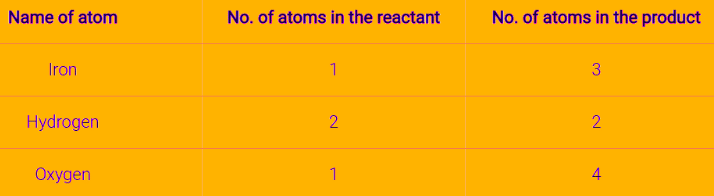Step 2:  Balance the atom which is maximum in number on either side of a chemical equation.
Start by balancing the number of oxygen atoms, which is the maximum on the right-hand side (RHS).
To balance oxygen, multiply the number of oxygen atoms on the left-hand side (LHS) by 4.
Fe + 4 × H2O → Fe3O4 + H2

Step 3: Balancing oxygen created an imbalance in the number of hydrogen atoms. There are now eight hydrogen atoms on the LHS and two on the RHS.
To balance hydrogen, multiply the number of hydrogen atoms on the RHS by 4.
Fe + 4 × H2O → Fe3O4 + 4 × H2

Step 4: There is only one iron atom on the LHS and three on the RHS.
To balance iron, multiply the number of iron atoms on the LHS by 3.
3 × Fe + 4 × H2O → Fe3O4 + 4 × H2

The equation is now balanced, and the same number of atoms of each element is present on both sides.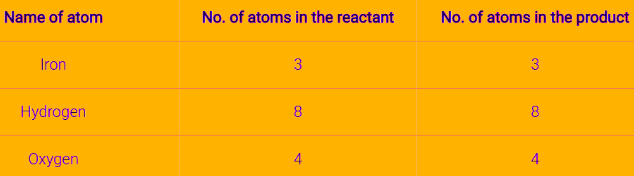After balancing, the above equation can be written as follows:
3Fe + 4H2O  Fe3O4 + 4H2

• To make a chemical equation more informative, it is important to include the physical state of the substances involved.
• The symbol (g) is used to represent a gaseous state, (l) for liquid state, (s) for solid state, and (aq) for an aqueous solution.
• The conditions under which the reaction takes place are also important to include in the chemical equation.
• These conditions can be written above and/or below the arrow in the chemical equation.
• Including this information can help provide a clearer understanding of the chemical reaction taking place.
• Example :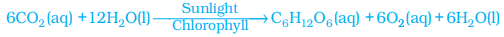• In this equation, (g) indicates that carbon dioxide is a gas, (l) indicates that water is a liquid, and (aq) indicates that glucose is dissolved in water. Sunlight and chlorophyll are specifically mentioned as the sources of energy used in the reaction.  This highlights the importance of these specific components in the photosynthesis process.

Question for Chapter Notes: Chemical Reactions & Equations
Try yourself:What is the balanced chemical equation for the reaction between hydrogen gas and oxygen gas to form water?

## Types of Chemical Reactions

### (1) Combination Reaction

It occurs when two or more reactants combine to form a single product.  or we say, such a reaction in which a single product is formed from two or more reactants is known as a combination reaction.

Example:

(i) Calcium oxide reacts vigorously with water to produce slaked lime (calcium hydroxide) releasing a large amount of heat.
CaO(s) + H2O(l) → Ca(OH)2 (aq) + Heat

(ii)  A solution of slaked lime produced by the reaction is used for white-washing walls. Calcium hydroxide reacts slowly with the carbon dioxide in the air to form a thin layer of calcium carbonate on the walls. Calcium carbonate is formed after two to three days of whitewashing and gives a shiny finish to the walls. It is interesting to note that the chemical formula for marble is also CaCO3.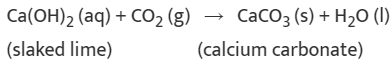(iii) Burning of Coal: When carbon is burnt in oxygen (air), carbon dioxide is formed. In this reaction, carbon is combined with oxygen.
C(s) + O2 (g) → CO(g)

### (2) Decomposition Reaction

A decomposition reaction occurs when one reactant breaks down into two or more products. This can be represented by the general equation: AB → A + B.

Example: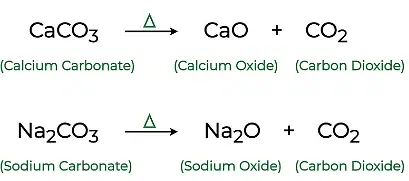Another example of it is, White silver chloride turns grey in sunlight. This is due to the decomposition of silver chloride into silver and chlorine by light.

Note: The above reactions are used in Black and White Photography.

### (3) Displacement Reaction

Displacement reaction is a chemical reaction in which a more reactive element displaces a less reactive element from its compound. Both metals and non-metals take part in displacement reactions.

Some Important Reactions as:
(i) Reaction of iron nails with copper sulphate solution.Fe (s) + CuSO4(aq) →  FeSO4(g) + Cu (s)
(ii)Reaction of lead with copper chloride solution.
Pb (s) + CuCl2(aq) →  PbCl2 (aq) + Cu (s)

Note:  Zinc and lead are more reactive elements than copper. They displace copper from its compounds.

Question for Chapter Notes: Chemical Reactions & Equations
Try yourself:Which of the following is an example of a displacement reaction?

### (4) Double Displacement Reaction

A double displacement reaction is a type of chemical reaction where two compounds react, and the positive ions (cation) and the negative ions (anion) of the two reactants switch places, forming two new compounds or products.

Example: When the solution of barium chloride reacts with the solution of sodium sulphate, white precipitate of barium sulphate is formed along with sodium chloride.
Na2(SO)4 (aq) + BaCl2 (aq) →  BaSO4 (s) + NaCl (aq)

Note: Double Displacement Reaction, in which precipitate is formed, is also known as precipitation reaction. Neutralisation reactions are also examples of double displacement reactions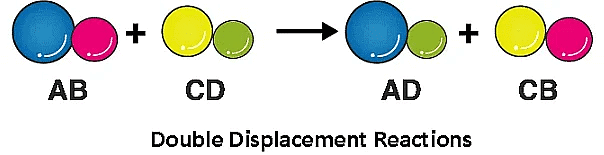### Some More Reactions:

(i) Exothermic Reaction:  An exothermic reaction is a type of chemical reaction in which energy is released from the reaction system into the surroundings, usually in the form of heat, light, or sound.

Example: Formation of Carbon dioxide
The chemical reaction can be depicted as:
C(s) + O2(g) → CO2(g) + Heat

(ii) Endothermic Reaction: An endothermic reaction is a type of chemical reaction in which energy is absorbed from the surroundings in the form of heat, light, or electricity.

Example: Photosynthesis
Plants absorb heat energy from sunlight to convert carbon dioxide and water into glucose and oxygen.

Reaction as:   6CO2 + 6H2O → C6H12O6 + 6O2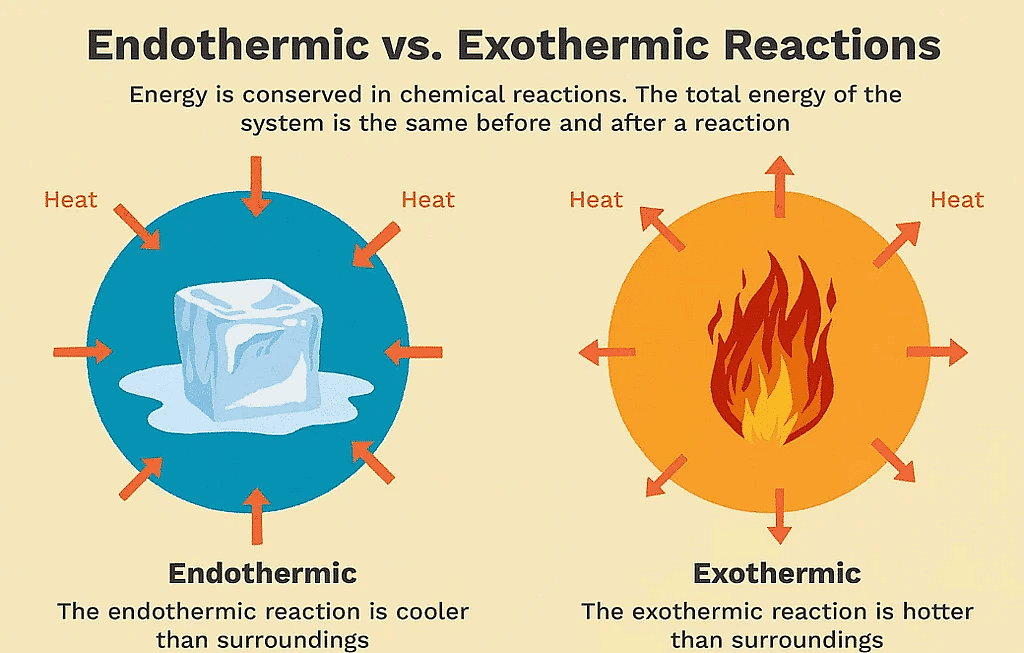Now lets discuss latest 2023  board questions  of this topic

Question for Chapter Notes: Chemical Reactions & Equations
Try yourself:Select the appropriate state symbols of the products given as X and Y in the following chemical equation by choosing the correct option from table given below:Zn(s) + H2SO4(l) → ZnSO4(X) + H2(Y)

## Oxidation and Reduction

Oxidation and reduction are two important chemical processes that involve the transfer of electrons between atoms or molecules.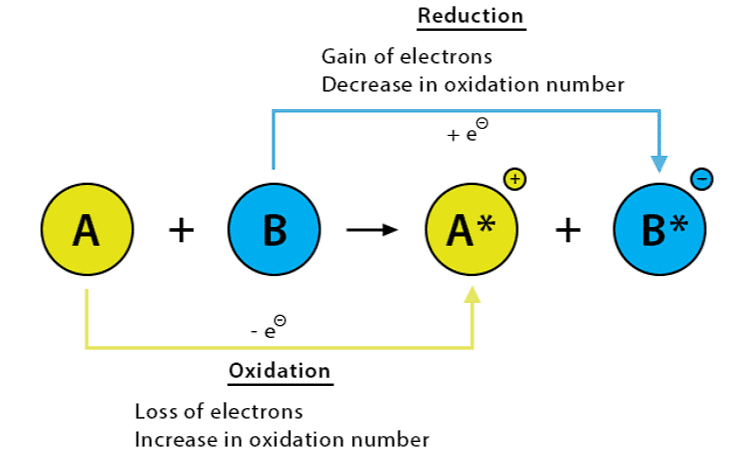(i) Oxidation is the process in which an atom, ion, or molecule loses one or more electrons. During oxidation, the oxidation state of the atom or molecule increases, since it becomes more positive or less negative.

Some examples of oxidation include the reaction of iron with oxygen to form rust and the reaction of glucose with oxygen in cellular respiration.

(ii) Reduction is the opposite process of oxidation, in which an atom, ion, or molecule gains one or more electrons.
Some examples of reduction include the reaction of silver ions with electrons to form silver metal, and the reaction of hydrogen ions with electrons to form hydrogen gas.

Question for Chapter Notes: Chemical Reactions & Equations
Try yourself:Which of the following statements is true about oxidation and reduction reactions?

### Redox Reaction, Oxidized Agent, Reducing Agent:

• Oxidation and reduction always occur together in a chemical reaction and are often referred to as redox reactions.
•  In a redox reaction, one substance is oxidized while another substance is reduced. The substance that is oxidized is called the reducing agent.
• It causes the reduction of another substance by donating electrons. The substance that is reduced is called the oxidizing agent. It causes the oxidation of another substance by accepting electrons.
• Example: In the reaction between zinc metal and hydrochloric acid, zinc is oxidized to form zinc ions, while hydrogen ions are reduced to form hydrogen gas:
Zn(s) + 2HCl(aq) → ZnCl2(aq) + H2(g)
• In this reaction, zinc is the reducing agent and hydrogen ions are the oxidizing agent.

## Effects of Oxidation Reaction on Everyday Life

### (i) Corrosion

The process of slow conversion of metals into undesirable compounds due to their reaction with oxygen, water, acids, gases etc. present in the atmosphere is called corrosion.

Rusting – Iron when reacts with oxygen and moisture forms a red substance called rust.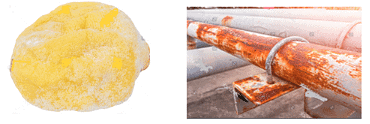### (ii) Rancidity

• The taste and odour of food materials containing fat and oil change when they are left exposed to air for a long time. This is called rancidity. It is caused due to oxidation of fat and oil present in food material.
• It can be prevented by using various methods such as adding antioxidants to the food materials, Storing food in an air-tight container and flushing out air with nitrogen.

Q1. What are chemical reactions?
Ans.
Chemical reactions are processes that involve the breaking of chemical bonds in one or more reactant molecules, followed by the rearrangement of atoms and the formation of new bonds to create one or more different molecules, known as products.

Q2. What is the difference between a chemical reaction and a chemical equation?
Ans.
A chemical reaction is a process that involves the breaking and formation of chemical bonds to create new substances, while a chemical equation is a symbolic representation of a chemical reaction that shows the reactants and products and their relative amounts. In other words, a chemical reaction is a physical process, whereas a chemical equation is a written description of that process.

Q3. What is the Law of Conservation of Mass?
Ans.
The Law of Conservation of Mass states that in any chemical reaction, the total mass of the reactants must equal the total mass of the products. This means that matter cannot be created or destroyed in a chemical reaction, only rearranged into different forms. This law is also known as the Law of Conservation of Matter.

Q4. How can you balance a chemical equation?
Ans.
To balance a chemical equation, you must ensure that the number of atoms of each element is the same on both the reactant and product sides of the equation. This is done by adjusting the coefficients in front of the chemical formulas until the number of atoms of each element is equal on both sides. It is important to note that you cannot change the subscripts within a chemical formula to balance an equation.

Q5. What are some common types of chemical reactions?
Ans.
Some common types of chemical reactions include combination reactions, decomposition reactions, displacement reactions, double displacement reactions, and combustion reactions. Combination reactions involve the combination of two or more reactants to form a single product, while decomposition reactions involve the breakdown of a single reactant into two or more products. Single displacement reactions involve the exchange of an ion or atom in a compound with a different ion or atom, while double displacement reactions involve the exchange of ions between two compounds. Combustion reactions involve the reaction of a substance with oxygen to produce heat and light.

The document Chemical Reactions & Equations Chapter Notes | Science Class 10 is a part of the Class 10 Course Science Class 10.
All you need of Class 10 at this link: Class 10

## Science Class 10

78 videos|509 docs|153 tests

## Science Class 10

78 videos|509 docs|153 testsExplore Courses for Class 10 examSignup to see your scores go up within 7 days! Learn & Practice with 1000+ FREE Notes, Videos & Tests.
10M+ students study on EduRev
Track your progress, build streaks, highlight & save important lessons and more!
Related Searches

,

,

,

,

,

,

,

,

,

,

,

,

,

,

,

,

,

,

,

,

,

;